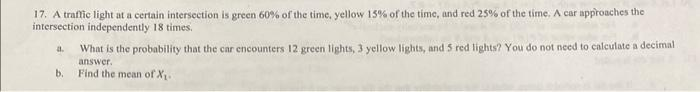Home / Expert Answers / Statistics and Probability / 17-a-traffic-light-at-a-certain-intersection-is-green-60-of-the-time-yellow-15-of-the-time-and-pa159

# (Solved): 17. A traffic light at a certain intersection is green 60% of the time, yellow 15% of the time, and ...17. A traffic light at a certain intersection is green of the time, yellow of the time, and red of the time. A car approaches the intersection independently 18 times. a. What is the probability that the car encounters 12 green lights, 3 yellow lights, and 5 red lights? You do not need to calculate a decimal answer. b. Find the mean of .

We have an Answer from Expert

Given data,

a. To calculate the probability that the car encounters 12 green lights, 3 yellow lights, and 5 red lights out of 18 total lights, we can use the concept of the multinomial probability.

The multinomial probability formula is:

Where:    is the probability of getting exactly k1 successes of type 1, k2 successes of type 2, k3 successes of type 3, ..., kn successes of type n. n is the total number of trials (in this case, the total number of lights).    are the desired number of successes of each type.    are the probabilities of success for each type.
In our case:
We have an Answer from Expert